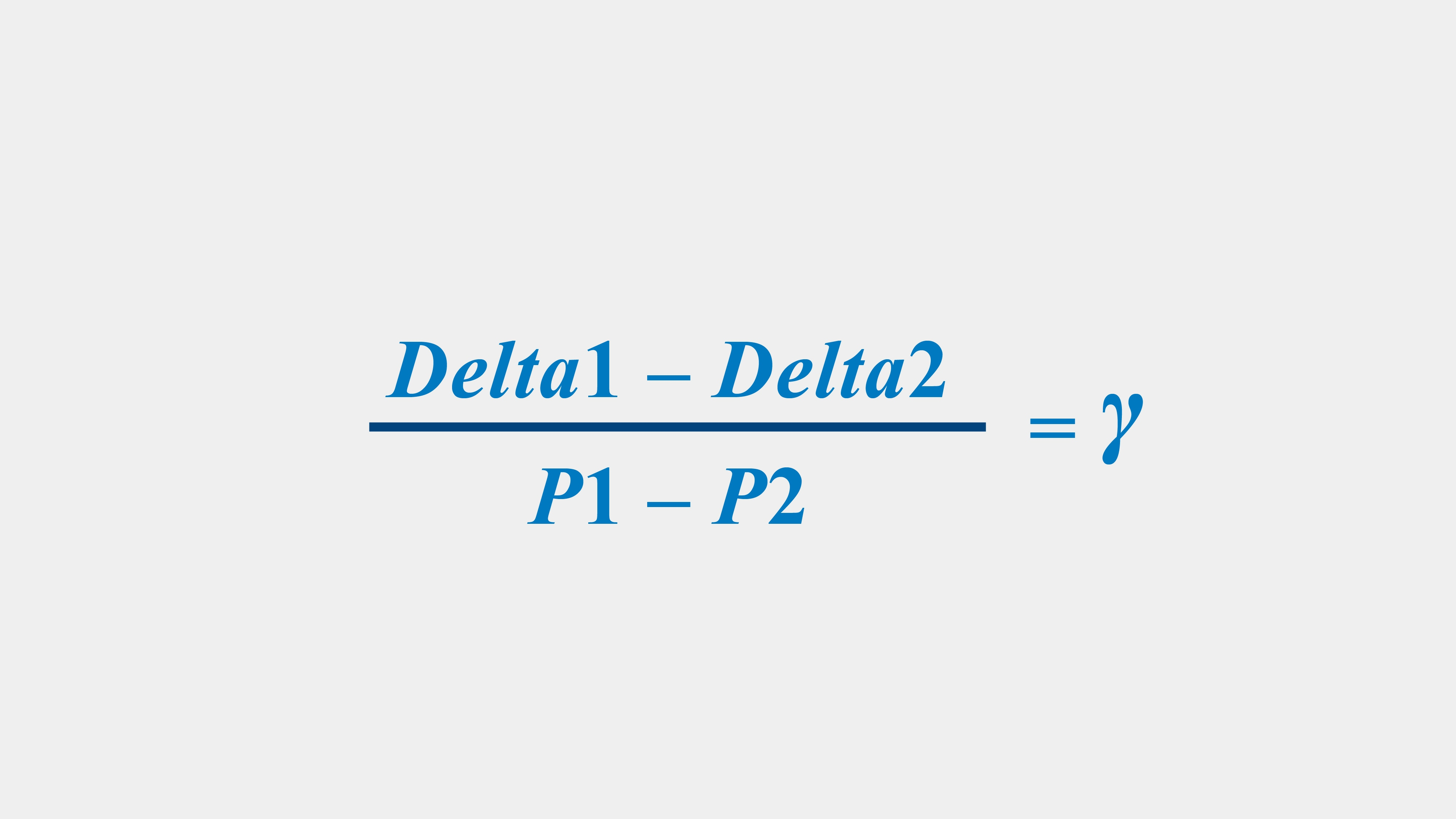## Stock Option Greeks: Gamma Explained

Gamma measures the rate of change of delta. Delta is a measure of a change in the price of the option (the premium). So therefore Gamma is measuring how fast the premium of the option is changing.

#### Calculating GammaImage Source: CME Group

#### An example

You are driving a motorcycle at 60 miles per hour (mph). If you increase your speed to 70 mph, you have accelerated by 10 miles per hour. If you think of speed as your delta, then the change in your speed is your gamma. In other words, gamma is your acceleration.

Sources: